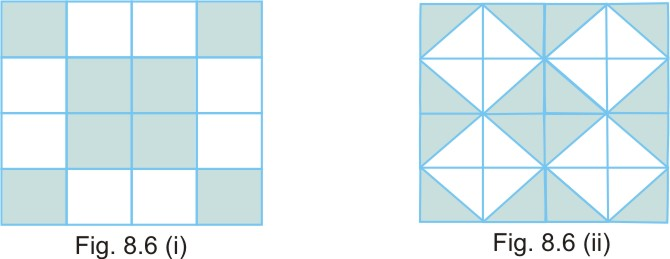#### In Fig. 8.6 (i) and Fig. 8.6 (ii), find the ratio of the area of the shaded portion to that of the whole figure:Assume the area of each small square is 1 square unit.

(i) Ratio of the area of the shaded portion to that of the whole figure in figure 1

(ii) Ratio of the area of the shaded portion to that of the whole figure in figure 2